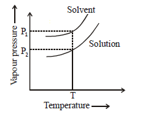The density of NH4OH solution is found to be 0.6 g/ml. It contains 35% by mass of NH4OH. The normality of the solution is

(1) 4.8 N

(2) 10 N

(3) 0.5 N

(4) 6 N

Concept Questions :-

Concentration Term and Numericals
High Yielding Test Series + Question Bank - NEET 2020

Difficulty Level:

The relative lowering of vapour caused by dissolving 71.3g of a substance in 1000g of water is 7.13x10-3. The molecular mass of substance is:-

1. 180

2. 18

3. 1.8

4. 360

Concept Questions :-

Relative Lowering of Vapour Pressure/ Vapour Pressure
High Yielding Test Series + Question Bank - NEET 2020

Difficulty Level:

Total vapour pressure of mixture of 1 mol A(p0A = 150 torr) and 2 mol B(P0B = 240 torr) is 200 torr. In this case:-

1. there is positive deviation from Raoult's law

2. there is negative deviation from Raoult's law

3. there is no deviation from Raoult's law

4. none of these

Concept Questions :-

Introduction/ Colligative properties
High Yielding Test Series + Question Bank - NEET 2020

Difficulty Level:

Which of the following aqueous solution should have the highest osmotic pressure?
(1) 0.011 M AICI
at 50°C
(2) 0.03 M NaCI at 25°C
(3) 0.012 M (NH
4)2SOat 25°C
(4) 0.03 M NaCI at 50°C

Concept Questions :-

Osmosis and Osmotic Pressure
High Yielding Test Series + Question Bank - NEET 2020

Difficulty Level:

50 g of antifreeze (ethylene glycol) is added to 200g water. What amount of ice will separate out at
-9.3°C. (Kf = 1.86 K Kg mol-1) :-

(1) 42 mg

(2) 42 g

(3) 38.71 g

(4) 38.71 mg

Concept Questions :-

Depression of Freezing Point
High Yielding Test Series + Question Bank - NEET 2020

Difficulty Level:

40 g NaOH (i=2) is dissolved in 1L volatile solvent vapour pressure  of this solution becomes equal to its solid phase at 300K. What is the freezing point of pure solvent.

(Density = 1g/mL) (kf=1.8 k kgmol-1)

1. 296.4 K

2. 303.6 K

3. 297.5 K

4. 302.4 K

Concept Questions :-

Depression of Freezing Point
High Yielding Test Series + Question Bank - NEET 2020

Difficulty Level:

P1 and P2 are vapour pressure of solvent and solution at temperature T. The solution is prepared by dissolving non-volatile solute in the same solvent. P1 =760 torr, P2 =684 mm Hg, Mole fraction of solvent in the solution will be:-(1) 0.1

(2) 0.2

(3) 0.9

(4) Can't be predicted

Concept Questions :-

Relative Lowering of Vapour Pressure/ Vapour Pressure
High Yielding Test Series + Question Bank - NEET 2020

Difficulty Level:

100 g solute is dissolved in 1400 g of solvent. Density of resultant solution is 1.5 g/mL. The ratio of its molarity and molality will be :-
(1)1.5

(2) 1.3

(3) 1.4

(4) 1.2

High Yielding Test Series + Question Bank - NEET 2020

Difficulty Level:

As scuba divers come towards water surface from underwater, solubility of gases in their blood :-
(1) Increases
(2) Decreases
(3) Firstly Increases then decrease
(4) Firstly Decreases then Increase

High Yielding Test Series + Question Bank - NEET 2020

Difficulty Level:

Solution A & B are formed by dissolving certain amount of glucose and sucrose in water. Vapour pressure of these solutions is 660 and 560 mm Hg respectively. Ratio of molality of solutions A & B will be :-

(1) 14/33
(2) 33/14
(3) 15/35
(4) 35/15